Math Courses / Course / Chapter

# Area Formulas of Triangles, Parallelograms & Trapezoids

Christian Killian, Laura Pennington
• Author
Christian Killian

Christian has a bachelor's degree in business administration, a master's degree in media communication and psychology, and credits toward a PhD in social psychology. They excel at math and science and enjoy explaining things to others. They are also OSHA 30 certified.

• Instructor
Laura Pennington

Laura received her Master's degree in Pure Mathematics from Michigan State University, and her Bachelor's degree in Mathematics from Grand Valley State University. She has 20 years of experience teaching collegiate mathematics at various institutions.

Learn how to find the areas of parallelograms, triangles, and trapezoids. Discover the similarities, differences, and relationships between these shapes. Updated: 01/28/2022

Show

#### How do you find the area of a parallelogram?

The formula for finding the area of a parallelogram is A = b x h, where b is the length of the base and h is the height. This is the same formula for area as that of a rectangle, since a parallelogram can be reconstructed to equal a rectangle shape.

#### How do the areas of triangles and parallelograms relate to each other?

The area of a triangle is half the length of its base times its height, while the area of a parallelogram is just the length of its base times its height. Two congruent triangles make up a parallelogram, and a parallelogram can be evenly divided into two congruent triangles.

Triangles are three-sided shapes that have three angles. Parallelograms are four-sided shapes (quadrilateral) where both sets of opposite sides are parallel. Trapezoids are four-sided shapes with only one set of opposite sides that are parallel. Parallelograms and trapezoids both have four internal angles, in contrast to the three internal angles of triangles.

The questions, "Is a triangle a parallelogram?" and "Is a triangle a trapezoid?" can be answered by referring to the defining characteristics of each shape.

### Is a Triangle a Parallelogram?

A triangle is not the same as a parallelogram, because each shape has a different number of sides and internal angles. Parallelograms also have two sets of parallel sides, where triangles have none. However, parallelograms can be broken down into triangles. A parallelogram is composed of two congruent triangles.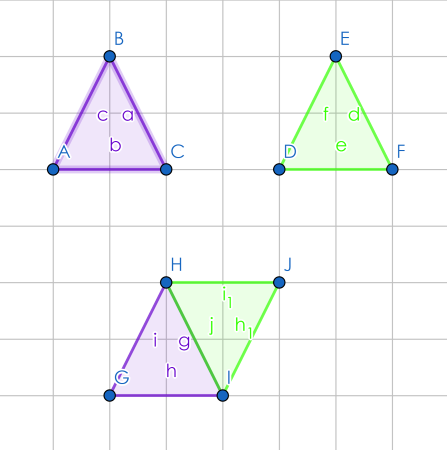To unlock this lesson you must be a Study.com Member.

## Three Different Shapes

Let's talk about shapes, three in particular! They are the triangle, the parallelogram, and the trapezoid. These three shapes are related in many ways, including their area formulas. The area of a two-dimensional shape is the amount of space inside that shape. Before we get to those relationships, let's take a moment to define each of these shapes and their area formulas.

Let's first look at parallelograms. A parallelogram is a four-sided, two-dimensional shape in which opposite sides are parallel and have equal length.

To find the area of a parallelogram, we simply multiply the base times the height.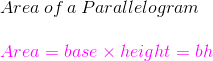Now, let's look at triangles. You've probably heard of a triangle. A triangle is a two-dimensional shape with three sides and three angles.

To find the area of a triangle, we take one half of its base multiplied by its height.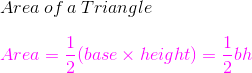Finally, let's look at trapezoids. A trapezoid is lesser known than a triangle, but still a common shape. A trapezoid is a four-sided, two-dimensional shape with two parallel sides. Trapezoids have two bases. Those are the sides that are parallel.

To find the area of a trapezoid, we multiply one half times the sum of the bases times the height.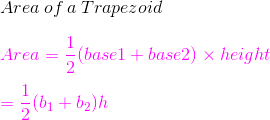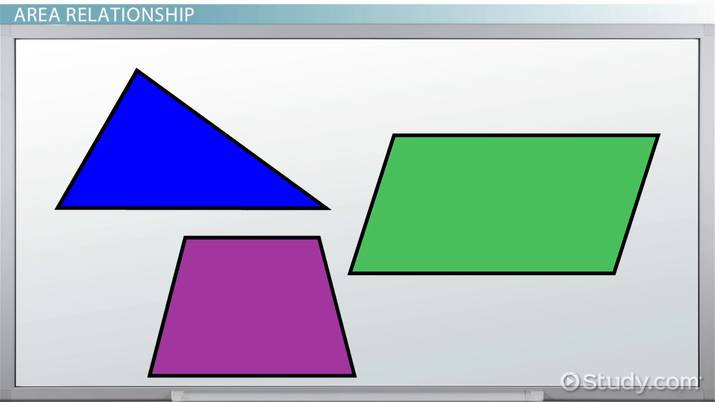An error occurred trying to load this video.

Try refreshing the page, or contact customer support.

Coming up next: Relationships Among Area Formulas of Various Quadrilaterals

### You're on a roll. Keep up the good work!

Replay
Your next lesson will play in 10 seconds
• 0:04 Three Different Shapes
• 1:12 Area Relationship
• 3:07 Lesson Summary
Save Timeline
Autoplay
Autoplay
Speed Speed

The formula to find the area of a triangle is A = 1/2 b x h, where:

• A is the area
• b is the length of the base of the triangle
• h is the height of the triangle, taken at its highest point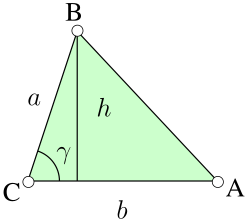The formula to find the area of a parallelogram can be derived from the formula to find the area of a triangle. Since a parallelogram is made up of two congruent triangles, the area of a parallelogram is the length of its base times its height (A = b x h), the same formula as used for a rectangle. Because a parallelogram is made up of two triangles with identical measurements, it has exactly twice the area of these triangles. Therefore, the '1/2' is not needed in the formula for finding the area of a parallelogram.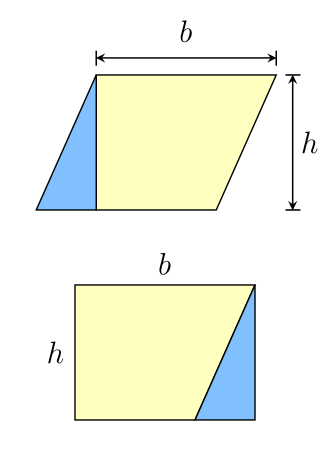### Examples of Parallelograms and Triangles

This section contains sample problems for finding the areas of parallelograms and triangles.

To unlock this lesson you must be a Study.com Member.

The formula for the area of a trapezoid is A = (a + b)/2 x h, where:

• a and b are the parallel sets of sides
• h is the height.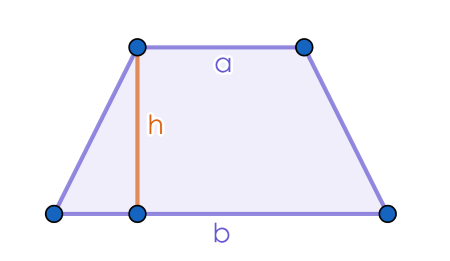### Examples of Triangles and Trapezoids

This section contains sample problems for finding the areas of trapezoids.

Example One: Find the area of a trapezoid with parallel side lengths of 6 feet and 8 feet, where the height of the trapezoid is 4 feet.

Remember that the formula for finding the area of a trapezoid is A = (a + b)/2 x h.

A = (a + b)/2 x h, where a = 6, b = 8, and h = 4

A = (6 + 8)/2 x 4

A = 14/2 x 4

A = 7 x 8

A = 56

The area of this trapezoid is 56 square feet.

To unlock this lesson you must be a Study.com Member.

This lesson covered the area formulas of triangles, parallelograms, and trapezoids. A triangle is a shape that has three sides and three internal angles, a parallelogram is a quadrilateral (four-sided shape) where both sets of opposite sides are parallel to each other, and a trapezoid is a quadrilateral where only one set of sides is parallel. Both parallelograms and trapezoids can be composed of congruent triangles, but trapezoids can also be composed of a set of three non-congruent triangles.

The formula to find the area of a triangle is A = 1/2 b x h, where b is the base of the triangle and h is the height of the triangle taken at its highest point. The formula to find the area of a parallelogram is the same as the formula to find the area of a rectangle, where A = b x h. The formula to find the area of a trapezoid is A = (a + b)/2 x h, where a and b are the parallel side lengths.

To unlock this lesson you must be a Study.com Member.

## Area Relationship

Alright! Now that we got all the definitions and formulas out of the way, let's look at how these three shapes' areas are related. To get started, let me ask you: do you like puzzles? That probably sounds odd, but as it turns out, we can create parallelograms using triangles or trapezoids as puzzle pieces. This fact will help us to illustrate the relationship between these shapes' areas.

Let's first look at the relationship between parallelograms and triangles. First, let's consider triangles and parallelograms. Notice that if we cut a parallelogram diagonally to divide it in half, we form two triangles, with the same base and height as the parallelogram.

We see that each triangle takes up precisely one half of the parallelogram. From this, we see that the area of a triangle is one half the area of a parallelogram, or the area of a parallelogram is two times the area of a triangle. By looking at a parallelogram as a puzzle put together by two equal triangle pieces, we have the relationship between the areas of these two shapes, like you can see in all these equations.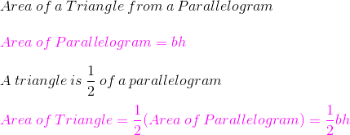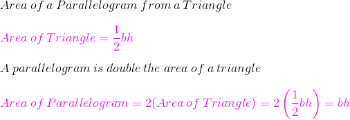Video Transcript

## Three Different Shapes

Let's talk about shapes, three in particular! They are the triangle, the parallelogram, and the trapezoid. These three shapes are related in many ways, including their area formulas. The area of a two-dimensional shape is the amount of space inside that shape. Before we get to those relationships, let's take a moment to define each of these shapes and their area formulas.

Let's first look at parallelograms. A parallelogram is a four-sided, two-dimensional shape in which opposite sides are parallel and have equal length.

To find the area of a parallelogram, we simply multiply the base times the height.Now, let's look at triangles. You've probably heard of a triangle. A triangle is a two-dimensional shape with three sides and three angles.

To find the area of a triangle, we take one half of its base multiplied by its height.Finally, let's look at trapezoids. A trapezoid is lesser known than a triangle, but still a common shape. A trapezoid is a four-sided, two-dimensional shape with two parallel sides. Trapezoids have two bases. Those are the sides that are parallel.

To find the area of a trapezoid, we multiply one half times the sum of the bases times the height.## Area Relationship

Alright! Now that we got all the definitions and formulas out of the way, let's look at how these three shapes' areas are related. To get started, let me ask you: do you like puzzles? That probably sounds odd, but as it turns out, we can create parallelograms using triangles or trapezoids as puzzle pieces. This fact will help us to illustrate the relationship between these shapes' areas.

Let's first look at the relationship between parallelograms and triangles. First, let's consider triangles and parallelograms. Notice that if we cut a parallelogram diagonally to divide it in half, we form two triangles, with the same base and height as the parallelogram.

We see that each triangle takes up precisely one half of the parallelogram. From this, we see that the area of a triangle is one half the area of a parallelogram, or the area of a parallelogram is two times the area of a triangle. By looking at a parallelogram as a puzzle put together by two equal triangle pieces, we have the relationship between the areas of these two shapes, like you can see in all these equations.To unlock this lesson you must be a Study.com Member.

### Register to view this lesson

Are you a student or a teacher?

Back

### Resources created by teachers for teachers

Over 30,000 video lessons & teaching resources‐all in one place.Video lessonsQuizzes & WorksheetsClassroom IntegrationLesson Plans

I would definitely recommend Study.com to my colleagues. It’s like a teacher waved a magic wand and did the work for me. I feel like it’s a lifeline.

Jennifer B.
TeacherCreate an account to start this course today
Used by over 30 million students worldwide